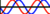# PHP TABLE TEMPLATES

These tables are all coded with PHP Loops. These table templates can be used for your table art, and by changing a few of the parameters, you can achieve different effects. Gradangles work well, as well as audioscopes. The Table is shown at a small size which you can easily change by changing the number of rows and columns in the code. The PHP code for each has highlighted sections in yellow as to what can be changed in the code. CCP these for your own personal use. When you CCP, take note of what you can change as the highlighted color can't be taken with you.
PLAIN
<?php
\$rowsDown=12;
\$row=1;
while(\$row<=\$rowsDown){
print("<tr>\n");
\$row++;
\$rowsAcross=5;
\$column=1;
while(\$column<=\$rowsAcross){
print("<td bgcolor=ffff00 align=center width=10 height=10>");
print("</td>");
\$column++;}
print("</tr>\n");}
print("</table>\n");
?>
ALTERNATE
<?php
\$rowsDown=12;
\$x=1;
while (\$x<=\$rowsDown){
echo "<tr>";
\$rowsAcross=1;
\$alt1="ff0000 width=10";
\$alt2="0000ff width=20";
\$alternating=\$alt1;
while(\$rowsAcross < 6){
\$rowsAcross = \$rowsAcross + 1;
if(\$alternating==\$alt1){
\$alternating=\$alt2; }
else {
\$alternating=\$alt1; } }
echo "</tr>";
\$x++; }
echo "</table>";
?>
CHECKERBOARD
<?php
\$rowsDown=12;
\$x=1;
while (\$x<=\$rowsDown){
print "<tr>";
\$rowsAcross=1;
\$alt1="ff0000";
\$alt2="0000ff";
while(\$rowsAcross < 8){
\$rowsAcross = \$rowsAcross + 1;
if ((\$x+\$rowsAcross)%2==\$alt1){
\$alternating=\$alt1;}
else {
\$alternating=\$alt2; }
print "<td height=10 width=10 bgcolor=\$alternating></td>"; }
print "</tr>";
\$x++; }
echo "</table>";
?>
HEXAGON
<?php
echo "<body bgcolor=000000>";
echo "<div align=center>";
//GLOBAL FUNCTION TO USE SAME VARIABLES
//IN MORE THAN ONE LOOP
function jump() {
GLOBAL \$tbl, \$cellR, \$end;}
echo "<table border=0 cellpadding=0 cellspacing=0><tr><td align=center>";
//LOOP #1 FOR TOP
\$width = 4;
while (\$width <=30) {
\$width = \$width + 2;
\$tbl = "<table border=0 cellpadding=0 cellspacing=0 bgcolor=0000ff><tr><td bgcolor=0000ff width=10></td><td bgcolor=ffff00 height=5 width=";
\$W = "\$width></td><td width=10></td>";
\$cellR = "<td bgcolor=ff0000 height=1 width=";
\$end = "</td></tr></table>";
echo \$tbl, \$W, \$cellR, \$W, \$end;
} //LOOP #2 FOR MIDDLE
\$times=30;
\$y=1;
\$width = 32;
while (\$y<=\$times){
echo \$tbl, \$W, \$cellR, \$W, \$end;
\$y++;}
//LOOP #3 FOR BOTTOM
\$width2 = 36;
while (\$width2 >= 4) {
\$width2 = \$width2 - 2;
\$W2 = "\$width2></td><td width=10></td>";
//CALL GLOBAL TO REUSE VARS
jump();
echo \$tbl, \$W2, \$cellR, \$W2, \$end;}
echo "</td></tr></table>";
echo "</div></body></html>";
?>AUDIOSCOPE WITH GIF
<?php echo "<bgsound src=\"song.midi\">";
echo "<html><body bgcolor=000000>";
function mirror() {
GLOBAL \$tbl, \$cell, \$end;}
echo "<table border=10 cellpadding=0 cellspacing=0 align=center bgcolor=ff00ff invertborder><tr><td align=center bgcolor=6600cc>";
\$width = 4;
while (\$width <= 80) {
\$width = \$width + 4;
\$tbl = "<table border=0 cellpadding=0 cellspacing=0 align=center><tr>";
\$cell = "<td bgcolor=ffff00 height=4 width=";
\$W="\$width><img src=http://www.lingolinda.com/clipart/scopes/jumpwave.gif height=4 width=";
\$AW= "\$width></td>";
\$end = "</td></tr></table>";
echo \$tbl, \$cell, \$W, \$AW, \$end;
} \$widthB = 82;
while (\$widthB>= 4) {
\$widthB = \$widthB - 4;
\$WB="\$widthB><img src=http://www.lingolinda.com/clipart/scopes/jumpwave.gif height=4 width=";
\$AWB= "\$widthB></td>";
mirror();
echo \$tbl, \$cell, \$WB, \$AWB, \$end;}
echo "</td></tr></table></body></html>";
?>